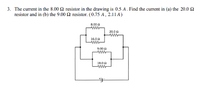# I am having some difficulties solving a combination circuits problem

EddyBenzen122
Homework Statement:
The current in the 8 - ohm resistor in the drawing is 5 A. Find the current in the 20 ohm and 9 - ohm resistor.
Relevant Equations:
V = IR
I'm having difficulties finding the current for a 9-ohm resistor only, not the 20-ohm

My attempt was to find the voltage of a 20ohm resistor which is V = (0.75A)(20-ohm) = 15V. I got the 0.75A by adding the currents of the two resistors (8-ohm and 16-ohm) together that were in parallel and it becomes the current that will flow through the 20-ohm resistor. After getting 15V, I don't know what to do next in order to find the current in the 9 - ohm resistor.

#### Attachments

•Screenshot 2021-09-14 214316.png
9.5 KB · Views: 44

Homework Helper
Gold Member
2022 Award
Homework Statement:: The current in the 8 - ohm resistor in the drawing is 5 A. Find the current in the 20 ohm and 9 - ohm resistor.
Relevant Equations:: V = IR

I'm having difficulties finding the current for a 9-ohm resistor only, not the 20-ohm

My attempt was to find the voltage of a 20ohm resistor which is V = (0.75A)(20-ohm) = 15V. I got the 0.75A by adding the currents of the two resistors (8-ohm and 16-ohm) together that were in parallel and it becomes the current that will flow through the 20-ohm resistor. After getting 15V, I don't know what to do next in order to find the current in the 9 - ohm resistor.
What other voltages can you determine?

EddyBenzen122
What other voltages can you determine?
4Volts from the parallel circuits with an 8-ohm and 16 - ohm

Homework Helper
Gold Member
2022 Award
4Volts from the parallel circuits with an 8-ohm and 16 - ohm
So what is the voltage across the ##9 \Omega ##|| ##18 \Omega## pair?

EddyBenzen122
So what is the voltage across the ##9 \Omega ##|| ##18 \Omega## pair?
that's what I'm looking for in order to find its current next.

Homework Helper
Gold Member
2022 Award
that's what I'm looking for in order to find its current next.
What is the relationship between the voltages:
- across the 9Ω|| 18Ω pair
- across the 8Ω|| 16Ω pair
- across the 20Ω resistor
?

EddyBenzen122
What is the relationship between the voltages:
- across the 9Ω|| 18Ω pair
the voltages are the same for both resistors
- across the 8Ω|| 16Ω pair
the voltages are the same for both resistors
- across the 20Ω resistor
?
the voltage is the sum of the currents for the 8-ohm resistor and the 10-ohm resistor multiplied by the 20-ohm resistor.

Homework Helper
Gold Member
2022 Award
the voltages are the same for both resistors
I didn't ask how the voltage across one of a pair compared with the voltage across the other of the pair. I asked about the relationship between the voltage across the 9Ω|| 18Ω pair (taken as a pair), the voltage across the 8Ω|| 16Ω pair (taken as a pair) and the voltage across the 20Ω resistor. Three voltages, one relationship.
the voltage is the sum of the currents for the 8-ohm resistor and the 10-ohm resistor multiplied by the 20-ohm resistor.
I have no idea how you arrive at that.

•sysprog
EddyBenzen122
I have no idea why you're asking me about the relationships between them, are you just giving me a hint?

Homework Helper
Gold Member
2022 Award
I have no idea why you're asking me about the relationships between them, are you just giving me a hint?
Of course I am giving a hint!

Call the voltage at the right of the 20 Ohm resistor 0.
So what is the voltage at the right of the 9||18 pair?

What is the voltage at the left of the 20 Ohm?
So what is the voltage at the left of the 8||16 pair?
So what is the voltage at the left of the 9||18 pair?

•sysprog
EddyBenzen122
Of course I am giving a hint!

Call the voltage at the right of the 20 Ohm resistor 0.
So what is the voltage at the right of the 9||18 pair?
What is the voltage at the left of the 20 Ohm?
So what is the voltage at the left of the 8||16 pair?
So what is the voltage at the left of the 9||18 pair?
K, i got the voltages: 4volts for 8||16 pair, 15V for 20ohm, and 19V for 9||18 pair.
Are they correct?

•sysprog
Homework Helper
Gold Member
2022 Award
K, i got the voltages: 4volts for 8||16 pair, 15V for 20ohm, and 19V for 9||18 pair.
Are they correct?
Yes.

•EddyBenzen122
sysprog
•EddyBenzen122 and berkeman
EddyBenzen122
Yes.
Thanks for helping me, I really appreciate it!

•sysprog